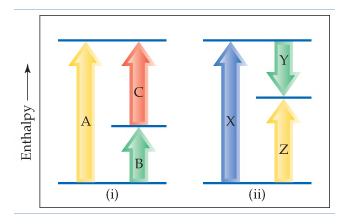# Problem: Consider the two diagrams below.Based on (ii), write an equation relating ΔHZ to the other enthalpy changes in the diagram.

###### FREE Expert Solution85% (448 ratings)###### Problem Details

Consider the two diagrams below.

Based on (ii), write an equation relating ΔHZ to the other enthalpy changes in the diagram.